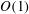Home

# The high-Reynolds-number asymptotic development of nonlinear equilibrium states in plane Couette flow

## Abstract

The relationship between nonlinear equilibrium solutions of the full Navier–Stokes equations and the high-Reynolds-number asymptotic vortex–wave interaction (VWI) theory developed for general shear flows by Hall & Smith (J. Fluid Mech., vol. 227, 1991, pp. 641–666) is investigated. Using plane Couette flow as a prototype shear flow, we show that all solutions having$\def \xmlpi #1{}\def \mathsfbi #1{\boldsymbol {\mathsf {#1}}}\let \le =\leqslant \let \leq =\leqslant \let \ge =\geqslant \let \geq =\geqslant \def \Pr {\mathit {Pr}}\def \Fr {\mathit {Fr}}\def \Rey {\mathit {Re}}O(1)$ wavenumbers converge to VWI states with increasing Reynolds number. The converged results here uncover an upper branch of VWI solutions missing from the calculations of Hall & Sherwin (J. Fluid Mech., vol. 661, 2010, pp. 178–205). For small values of the streamwise wavenumber, the converged lower-branch solutions take on the long-wavelength state of Deguchi, Hall & Walton (J. Fluid Mech., vol. 721, 2013, pp. 58–85) while the upper-branch solutions are found to be quite distinct, with new states associated with instabilities of jet-like structures playing the dominant role. Between these long-wavelength states, a complex ‘snaking’ behaviour of solution branches is observed. The snaking behaviour leads to complex ‘entangled’ states involving the long-wavelength states and the VWI states. The entangled states exhibit different-scale fluid motions typical of those found in shear flows.

## References

Hide All
MathJax
MathJax is a JavaScript display engine for mathematics. For more information see http://www.mathjax.org.

# The high-Reynolds-number asymptotic development of nonlinear equilibrium states in plane Couette flow

## Metrics

### Full text viewsFull text views reflects the number of PDF downloads, PDFs sent to Google Drive, Dropbox and Kindle and HTML full text views.

Total number of HTML views: 0
Total number of PDF views: 0 *Loading metrics...

### Abstract viewsAbstract views reflect the number of visits to the article landing page.

Total abstract views: 0 *Loading metrics...

* Views captured on Cambridge Core between <date>. This data will be updated every 24 hours.

Usage data cannot currently be displayed